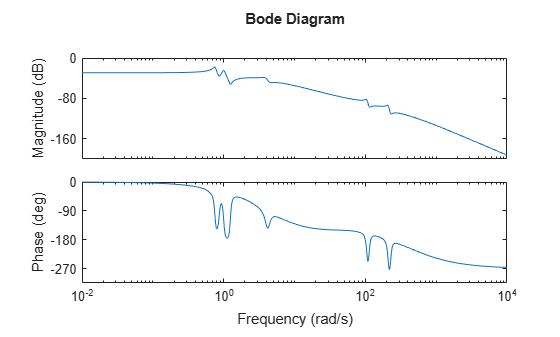# freqsep

Slow-fast decomposition

## Syntax

``````[G1,G2] = freqsep(G,fcut)``````
``````[G1,G2] = freqsep(G,[fmin,fmax])``````
``````[G1,G2] = freqsep(G,___,Name=Value)``````
``[G1,G2,info] = freqsep(___)``

## Description

example

``````[G1,G2] = freqsep(G,fcut)``` decomposes a linear dynamic system into slow and fast components around the specified cutoff frequency. The decomposition is `G` = `G1` + `G2`, where `G1` contains all modes with natural frequency less than `fcut`.```
``````[G1,G2] = freqsep(G,[fmin,fmax])``` computes the decomposition where `G1` contains all modes with natural frequency fmin ≤ ωn ≤ fmax and `G2` contains the remaining modes. (since R2023b)```

example

``````[G1,G2] = freqsep(G,___,Name=Value)``` specifies additional options to control accuracy. (since R2023b)```
````[G1,G2,info] = freqsep(___)` returns a structure `info` containing the block-diagonalizing transformation matrices. (since R2023b)```

## Examples

collapse all

```load numdemo Pd bode(Pd)````Pd` has four complex poles and one real pole. The Bode plot shows a resonance around 210 rad/s and a higher-frequency resonance below 10,000 rad/s.

Decompose this model around 1000 rad/s to separate these two resonances.

```[Gs,Gf] = freqsep(Pd,10^3); bode(Pd,Gs,Gf) legend('original','slow','fast','Location','Southwest')```The Bode plot shows that the slow component, `Gs`, contains only the lower-frequency resonance. This component also matches the DC gain of the original model. The fast component, `Gf`, contains the higher-frequency resonances and matches the response of the original model at high frequencies. The sum of the two components `Gs+Gf` yields the original model.

Decompose a model into slow and fast components between poles that are closely spaced.

The following system includes a real pole and a complex pair of poles that are all close to s = -2.

`G = zpk(-.5,[-1.9999 -2+1e-4i -2-1e-4i],10);`

Try to decompose the model about 2 rad/s, so that the slow component contains the real pole and the fast component contains the complex pair.

`[Gs,Gf] = freqsep(G,2);`
```Warning: One or more fast modes could not be separated from the slow modes. To force separation, relax the accuracy constraint by increasing the "SepTol" factor (see "freqsepOptions" for details). ```

These poles are too close together for `freqsep` to separate. Increase the relative tolerance to allow the separation.

`[Gs,Gf] = freqsep(G,2,SepTol=5e10);`

Now `freqsep` successfully separates the dynamics.

`slowpole = pole(Gs)`
```slowpole = -1.9999 ```
`fastpole = pole(Gf)`
```fastpole = 2×1 complex -2.0000 + 0.0001i -2.0000 - 0.0001i ```

This example shows how to decompose a model and retain the modes in a specified frequency range using `freqsep`.

Load the model `Gms` and examine its frequency response.

```load modeselect Gms bodeplot(Gms)```Use `freqsep` to retain the dynamics in the frequency range 0.1 rad/s to 50 rad/s.

`[G1,G2] = freqsep(Gms,[0.1,50]);`

In this decomposition, the output `G1` contains all poles with natural frequency in the range `[0.1,50]` and `G2` contains the remaining poles.

```bodeplot(Gms,G1,G2) legend```## Input Arguments

collapse all

Dynamic system to decompose, specified as a numeric LTI model, such as a `ss` or `tf` model.

Cutoff frequency for fast-slow decomposition, specified as a positive scalar. The output `G1` contains all poles with natural frequency less than `fcut`. The output `G2` contains all poles with natural frequency greater than or equal to `fcut`.

Since R2023b

Frequency range for decomposition, specified as a two-element vector. The output `G1` contains all poles with natural frequency in the range `[fmin,fmax]`. The output `G2` contains the remaining poles.

### Name-Value Arguments

Specify optional pairs of arguments as `Name1=Value1,...,NameN=ValueN`, where `Name` is the argument name and `Value` is the corresponding value. Name-value arguments must appear after other arguments, but the order of the pairs does not matter.

Example: `[G1,G2] = freqsep(G,2,SepTol=100)`

Since R2023b

Accuracy loss factor for slow-fast decomposition, specified as a nonnegative scalar value. `freqresp` ensures that the frequency responses of the original system, `G`, and the sum of the decomposed systems `G1+G2`, differ by no more than `SepTol` times the absolute accuracy of the computed value of `G(s)`. Increasing `SepTol` helps separate modes straddling the slow/fast boundary at the expense of accuracy.

## Output Arguments

collapse all

Slow dynamics of the decomposed system, returned as a numeric LTI model of the same type as `G`. `G1` contains all poles of `G` with natural frequency less than `fcut`, and is such that `G` = `G1` + `G2`.

Fast dynamics of the decomposed system, returned as a numeric LTI model of the same type as `G`. `Gf` contains all poles of `G` with natural frequency greater than or equal to `fcut`, and is such that `G` = `G1` + `G2`.

Since R2023b

FieldDescription
`TL`Left-side matrix of the block-diagonalizing transformation, returned as a matrix with dimensions Nx-by-Nx, where Nx is the number of states in the model G.
`TR`Right-side matrix of the block-diagonalizing transformation, returned as a matrix with dimensions Nx-by-Nx, where Nx is the number of states in the model G.

The algorithm transforms the state-space realization (A, B, C, D) of the model `G` to

`$\begin{array}{ccc}{T}_{L}A{T}_{R}=\left(\begin{array}{cc}{A}_{1}& 0\\ 0& {A}_{2}\end{array}\right),& {T}_{L}B=\left(\begin{array}{c}{B}_{1}\\ {B}_{2}\end{array}\right),& C{T}_{R}=\left(\begin{array}{cc}{C}_{1}& {C}_{2}\end{array}\right)\end{array}$`

The function returns an empty value `[]` for this argument when the input model `G` is not a state-space model.

Model Reducer